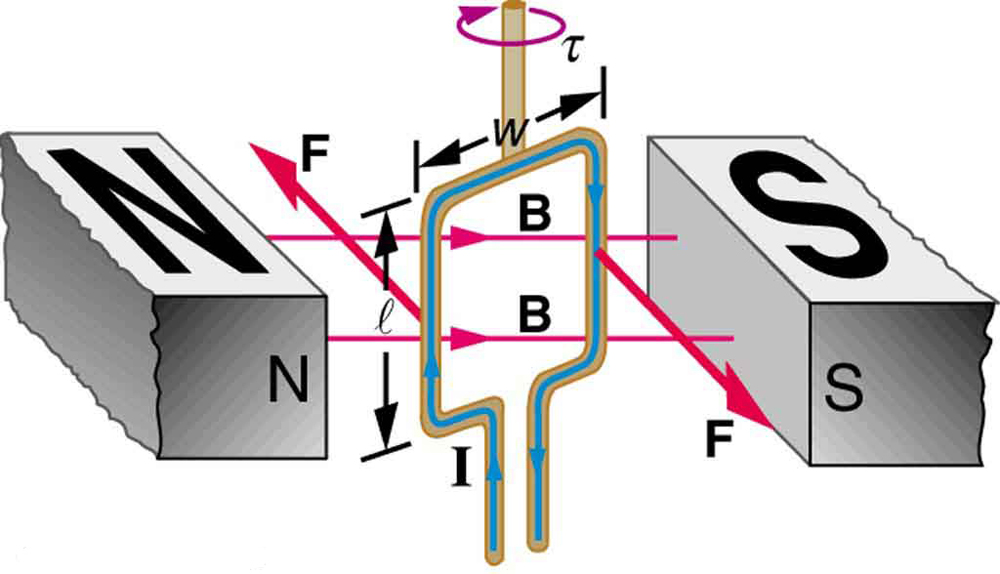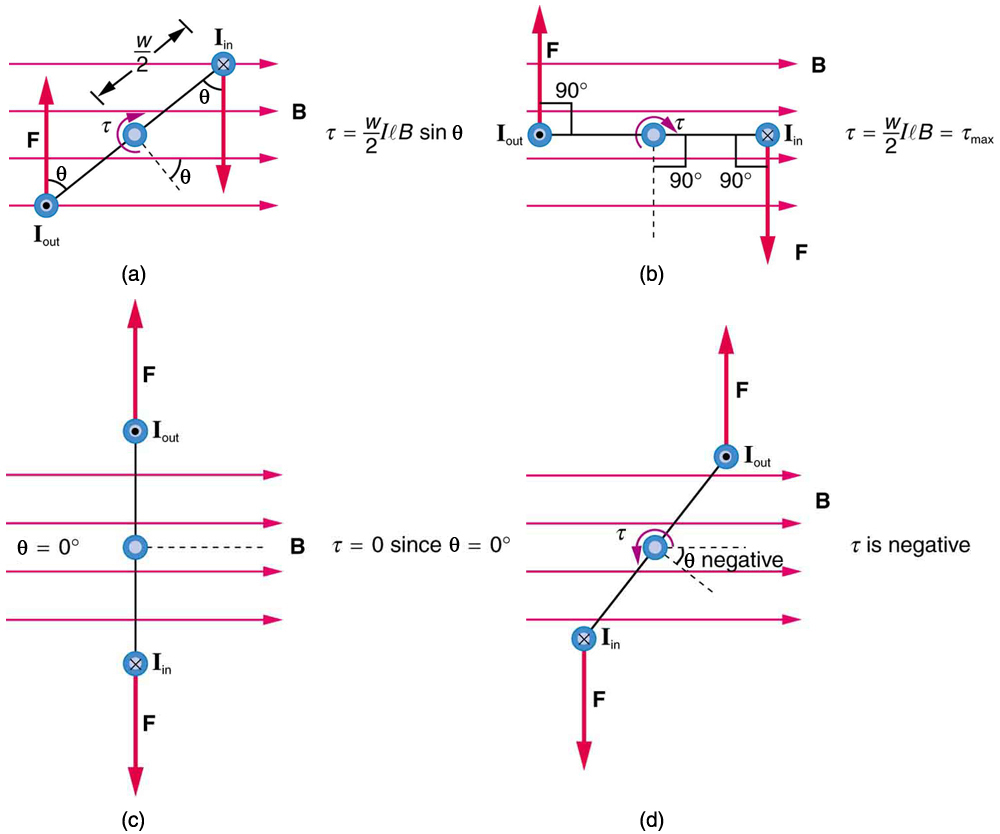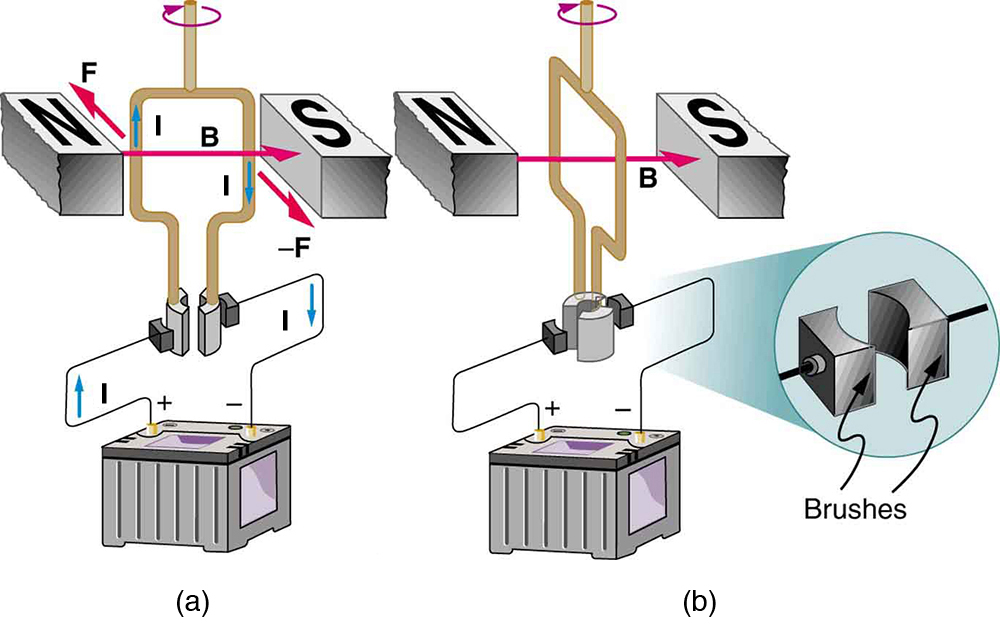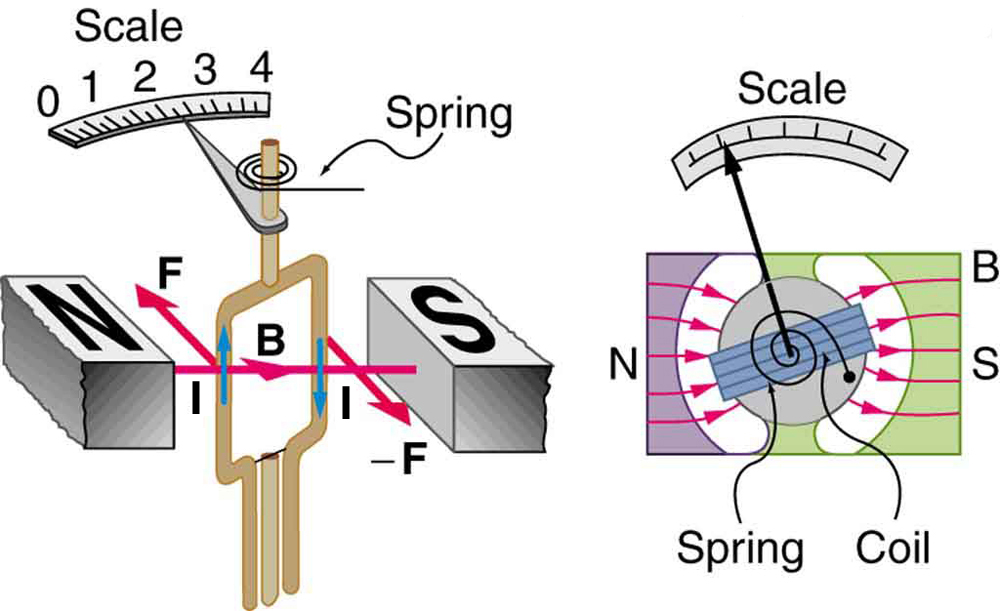Chapter 22 Magnetism

# 22.8 Torque on a Current Loop: Motors and Meters

Torque on a Current Loop: Motors and Meters
• Describe how motors and meters work in terms of torque on a current loop.
• Calculate the torque on a current-carrying loop in a magnetic field.

Motors are the most common application of magnetic force on current-carrying wires. Motors have loops of wire in a magnetic field. When current is passed through the loops, the magnetic field exerts torque on the loops, which rotates a shaft. Electrical energy is converted to mechanical work in the process. (See [link].)Torque on a current loop. A current-carrying loop of wire attached to a vertically rotating shaft feels magnetic forces that produce a clockwise torque as viewed from above.

Let us examine the force on each segment of the loop in [link] to find the torques produced about the axis of the vertical shaft. (This will lead to a useful equation for the torque on the loop.) We take the magnetic field to be uniform over the rectangular loop, which has width ww and height ll. First, we note that the forces on the top and bottom segments are vertical and, therefore, parallel to the shaft, producing no torque. Those vertical forces are equal in magnitude and opposite in direction, so that they also produce no net force on the loop. [link] shows views of the loop from above. Torque is defined as τ=rFsinθτ=rFsinθ size 12{τ= ital “rF””sin”θ} {}, where FF size 12{F} {} is the force, rr is the distance from the pivot that the force is applied, and θθ is the angle between rr and FF. As seen in [link](a), right hand rule 1 gives the forces on the sides to be equal in magnitude and opposite in direction, so that the net force is again zero. However, each force produces a clockwise torque. Since r=w/2r=w/2, the torque on each vertical segment is (w/2)Fsinθ(w/2)Fsinθ, and the two add to give a total torque.

τ=w2Fsinθ+w2Fsinθ=wFsinθτ=w2Fsinθ+w2Fsinθ=wFsinθ size 12{τ= { {w} over {2} } F”sin”θ+ { {w} over {2} } F”sin”θ= ital “wF””sin”θ} {}Top views of a current-carrying loop in a magnetic field. (a) The equation for torque is derived using this view. Note that the perpendicular to the loop makes an angle θθ size 12{θ} {} with the field that is the same as the angle between w/2w/2 size 12{w/2} {} and FF size 12{F} {}. (b) The maximum torque occurs when θθ size 12{θ} {} is a right angle and sinθ=1sinθ=1 size 12{“sin”θ=1} {}. (c) Zero (minimum) torque occurs when θθ size 12{θ} {} is zero and sinθ=0sinθ=0. (d) The torque reverses once the loop rotates past θ=0θ=0.

Now, each vertical segment has a length ll size 12{l} {} that is perpendicular to BB size 12{B} {}, so that the force on each is F=IlBF=IlB size 12{F= ital “IlB”} {}. Entering FF size 12{F} {} into the expression for torque yields

τ=wIlBsinθ.τ=wIlBsinθ. size 12{τ= ital “wIlB””sin”θ} {}

If we have a multiple loop of NN size 12{N} {} turns, we get NN size 12{N} {} times the torque of one loop. Finally, note that the area of the loop is A=wlA=wl size 12{A= ital “wl”} {}; the expression for the torque becomes

τ=NIABsinθ.τ=NIABsinθ. size 12{τ= ital “NIAB””sin”θ} {}

This is the torque on a current-carrying loop in a uniform magnetic field. This equation can be shown to be valid for a loop of any shape. The loop carries a current II size 12{I} {}, has NN size 12{N} {} turns, each of area AA size 12{A} {}, and the perpendicular to the loop makes an angle θθ size 12{θ} {} with the field BB size 12{B} {}. The net force on the loop is zero.

Calculating Torque on a Current-Carrying Loop in a Strong Magnetic Field

Find the maximum torque on a 100-turn square loop of a wire of 10.0 cm on a side that carries 15.0 A of current in a 2.00-T field.

Strategy

Torque on the loop can be found using τ=NIABsinθτ=NIABsinθ size 12{τ= ital “NIAB””sin”θ} {}. Maximum torque occurs when θ=90ºθ=90º and sinθ=1sinθ=1 size 12{“sin”θ=1} {}.

Solution

For sinθ=1sinθ=1 size 12{“sin”θ=1} {}, the maximum torque is

τmax=NIAB.τmax=NIAB. size 12{τ rSub { size 8{“max”} } = ital “NIAB”} {}

Entering known values yields

τmax=10015.0 A0.100 m22.00 T=30.0 N⋅m.τmax=10015.0 A0.100 m22.00 T=30.0 N⋅m.alignl { stack {
size 12{τ rSub { size 8{“max”} } = left (“100” right ) left (“15” “.” 0″ A” right ) left (0 “.” “100”” m” rSup { size 8{2} } right ) left (2 “.” “00”” T” right )} {} #
” “=”30” “.” “0 N” cdot m “.” {}
} } {}

Discussion

This torque is large enough to be useful in a motor.

The torque found in the preceding example is the maximum. As the coil rotates, the torque decreases to zero at θ=0θ=0 size 12{θ=0} {}. The torque then reverses its direction once the coil rotates past θ=0θ=0 size 12{θ=0} {}. (See [link](d).) This means that, unless we do something, the coil will oscillate back and forth about equilibrium at θ=0θ=0 size 12{θ=0} {}. To get the coil to continue rotating in the same direction, we can reverse the current as it passes through θ=0θ=0 size 12{θ=0} {} with automatic switches called brushes. (See [link].)(a) As the angular momentum of the coil carries it through θ=0θ=0 size 12{θ=0} {}, the brushes reverse the current to keep the torque clockwise. (b) The coil will rotate continuously in the clockwise direction, with the current reversing each half revolution to maintain the clockwise torque.

Meters, such as those in analog fuel gauges on a car, are another common application of magnetic torque on a current-carrying loop. [link] shows that a meter is very similar in construction to a motor. The meter in the figure has its magnets shaped to limit the effect of θθ size 12{θ} {} by making BB size 12{B} {} perpendicular to the loop over a large angular range. Thus the torque is proportional to II size 12{I} {} and not θθ size 12{θ} {}. A linear spring exerts a counter-torque that balances the current-produced torque. This makes the needle deflection proportional to II size 12{I} {}. If an exact proportionality cannot be achieved, the gauge reading can be calibrated. To produce a galvanometer for use in analog voltmeters and ammeters that have a low resistance and respond to small currents, we use a large loop area AA size 12{A} {}, high magnetic field BB size 12{B} {}, and low-resistance coils.Meters are very similar to motors but only rotate through a part of a revolution. The magnetic poles of this meter are shaped to keep the component of BB size 12{B} {} perpendicular to the loop constant, so that the torque does not depend on θθ size 12{θ} {} and the deflection against the return spring is proportional only to the current II size 12{I} {}.

# Section Summary

• The torque ττ size 12{τ} {} on a current-carrying loop of any shape in a uniform magnetic field. is
τ=NIABsinθ,τ=NIABsinθ, size 12{τ= ital “NIAB””sin”θ} {}

where NN size 12{N} {} is the number of turns, II size 12{I} {} is the current, AA size 12{A} {} is the area of the loop, BB size 12{B} {} is the magnetic field strength, and θθ size 12{θ} {} is the angle between the perpendicular to the loop and the magnetic field.

# Conceptual Questions

Draw a diagram and use RHR-1 to show that the forces on the top and bottom segments of the motor’s current loop in [link] are vertical and produce no torque about the axis of rotation.

# Problems & Exercises

(a) By how many percent is the torque of a motor decreased if its permanent magnets lose 5.0% of their strength? (b) How many percent would the current need to be increased to return the torque to original values?

(a) τ τ size 12{” τ”} {} decreases by 5.00% if B decreases by 5.00%

(b) 5.26% increase

(a) What is the maximum torque on a 150-turn square loop of wire 18.0 cm on a side that carries a 50.0-A current in a 1.60-T field? (b) What is the torque when θθ size 12{θ} {} is 10.9º?10.9º? size 12{“10” “.” 9°?} {}

Find the current through a loop needed to create a maximum torque of 9.00 N⋅m.9.00 N⋅m. size 12{9 “.” “00”`N cdot m “.” } {} The loop has 50 square turns that are 15.0 cm on a side and is in a uniform 0.800-T magnetic field.

10.0 A

Calculate the magnetic field strength needed on a 200-turn square loop 20.0 cm on a side to create a maximum torque of 300 N⋅m300 N⋅m size 12{3″00″`N cdot m} {} if the loop is carrying 25.0 A.

Since the equation for torque on a current-carrying loop is τ=NIABsinθτ=NIABsinθ size 12{τ= ital “NIAB””sin”θ} {}, the units of N⋅mN⋅m size 12{N cdot m} {} must equal units of A⋅m2TA⋅m2T size 12{A cdot m rSup { size 8{2} } `T} {}. Verify this.

A⋅m2⋅T=A⋅m2NA⋅m=N⋅mA⋅m2⋅T=A⋅m2NA⋅m=N⋅m size 12{A cdot m rSup { size 8{2} } cdot T=A cdot m rSup { size 8{2} } left ( { {N} over {A cdot m} } right )=N cdot m} {}.

(a) At what angle θθ size 12{θ} {} is the torque on a current loop 90.0% of maximum? (b) 50.0% of maximum? (c) 10.0% of maximum?

A proton has a magnetic field due to its spin on its axis. The field is similar to that created by a circular current loop 0.650×10−15m0.650×10−15m size 12{0 “.” “650” times “10” rSup { size 8{ – “15”} } `m} {} in radius with a current of 1.05×104A1.05×104A size 12{1 “.” “05” times “10” rSup { size 8{4} } `A} {} (no kidding). Find the maximum torque on a proton in a 2.50-T field. (This is a significant torque on a small particle.)

3.48×10−26N⋅m3.48×10−26N⋅m size 12{3 “.” “48” times “10” rSup { size 8{ – “26”} } `N cdot m} {}

(a) A 200-turn circular loop of radius 50.0 cm is vertical, with its axis on an east-west line. A current of 100 A circulates clockwise in the loop when viewed from the east. The Earth’s field here is due north, parallel to the ground, with a strength of 3.00×10−5T3.00×10−5T size 12{3 “.” “00” times “10” rSup { size 8{ – 5} } `T} {}. What are the direction and magnitude of the torque on the loop? (b) Does this device have any practical applications as a motor?

Repeat [link], but with the loop lying flat on the ground with its current circulating counterclockwise (when viewed from above) in a location where the Earth’s field is north, but at an angle 45.0º45.0º size 12{“45” “.” 0°} {} below the horizontal and with a strength of 6.00×10−5T6.00×10−5T size 12{6 “.” “00” times “10” rSup { size 8{ – 5} } `T} {}.

(a) 0.666 N⋅m0.666 N⋅m size 12{0 “.” “666”`N cdot m} {} west

(b) This is not a very significant torque, so practical use would be limited. Also, the current would need to be alternated to make the loop rotate (otherwise it would oscillate).

## Glossary

motor
loop of wire in a magnetic field; when current is passed through the loops, the magnetic field exerts torque on the loops, which rotates a shaft; electrical energy is converted to mechanical work in the process
meter
common application of magnetic torque on a current-carrying loop that is very similar in construction to a motor; by design, the torque is proportional to II and not θθ, so the needle deflection is proportional to the current